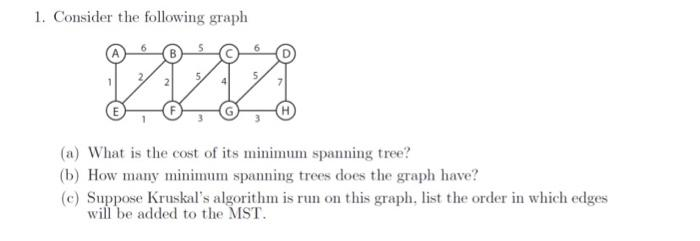# Question 1. Consider the following graph 5 2 (a) What is the cost of its minimum spanning tree? (b) How many minimum spanning trees does the graph have? (c) Suppose Kruskal's algorithm is run on this graph, list the order in which edges will be added to the MST.JECJ1K The Asker · Computer ScienceTranscribed Image Text: 1. Consider the following graph 5 2 (a) What is the cost of its minimum spanning tree? (b) How many minimum spanning trees does the graph have? (c) Suppose Kruskal's algorithm is run on this graph, list the order in which edges will be added to the MST.
More
Transcribed Image Text: 1. Consider the following graph 5 2 (a) What is the cost of its minimum spanning tree? (b) How many minimum spanning trees does the graph have? (c) Suppose Kruskal's algorithm is run on this graph, list the order in which edges will be added to the MST.